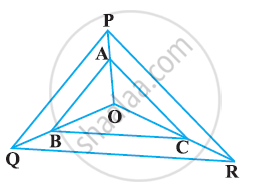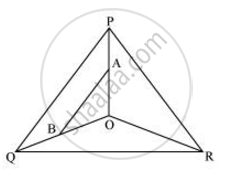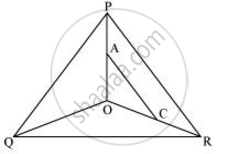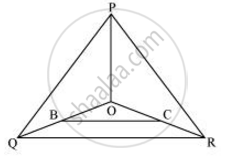Share

# In the following figure, A, B and C are points on OP, OQ and OR respectively such that AB || PQ and AC || PR. Show that BC || QR. - CBSE Class 10 - Mathematics

#### Question

In the following figure, A, B and C are points on OP, OQ and OR respectively such that AB || PQ and AC || PR. Show that BC || QR.#### SolutionIn Δ POQ, AB || PQ

:.(OA)/(AP) = (OB)/(BQ)   (basic proportionality theorem)  (i)In ΔPOR, AC||PR

:.(OA)/(AP) = (OC)/(CR)  (By basic proportionality theorem)  (ii)

From i and ii, we obtain

(OB)/(BQ) = (OC)/(CR)

:. BC || OQ (By Converse of basic proportionality theorem)Is there an error in this question or solution?

#### APPEARS IN

NCERT Solution for Mathematics Textbook for Class 10 (2019 to Current)
Chapter 6: Triangles
Ex. 6.20 | Q: 6 | Page no. 129

#### Video TutorialsVIEW ALL 

Solution In the following figure, A, B and C are points on OP, OQ and OR respectively such that AB || PQ and AC || PR. Show that BC || QR. Concept: Similarity of Triangles.
S